# Suppose than an object that is originally at room temperature of 30 Celsius is placed in a freezer. The temperature T (x) of the object can be approximated by the model T (x) = 360 / xˆ2 + 4x + 12, where x is the time in hours after the object is placed in the freezer.a) What is the horizontal asymptote of the graph of this function and what does it represent in the context of this problem?b) A chemist needs a compound cooled to less than 5 Celsius. Determine the amount of time required for the compound to cool so that its temperature is less than 5 Celsius.

Question
5 views

Suppose than an object that is originally at room temperature of 30 Celsius is placed in a freezer. The temperature T (x) of the object can be approximated by the model T (x) = 360 / xˆ2 + 4x + 12, where x is the time in hours after the object is placed in the freezer.

a) What is the horizontal asymptote of the graph of this function and what does it represent in the context of this problem?

b) A chemist needs a compound cooled to less than 5 Celsius. Determine the amount of time required for the compound to cool so that its temperature is less than 5 Celsius.

check_circle

Step 1

Given: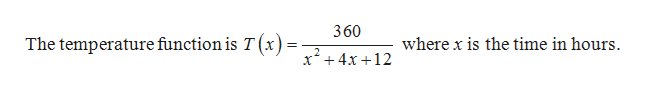help_outlineImage Transcriptionclose360 where x is the time in hours The temperature function is T(x)= 2 x4x12 fullscreen
Step 2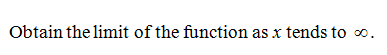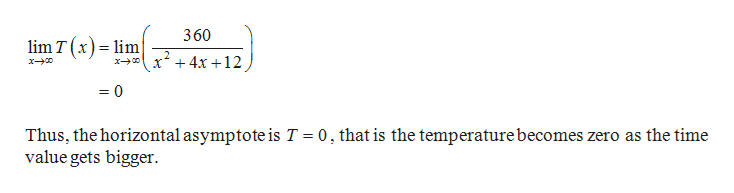help_outlineImage Transcriptionclose360 lim T (x)lm x4x12 = 0 Thus, the horizontal asymptote is T 0, that is the temperature becomes zero as the time value gets bigger fullscreen
Step 3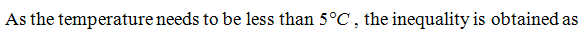...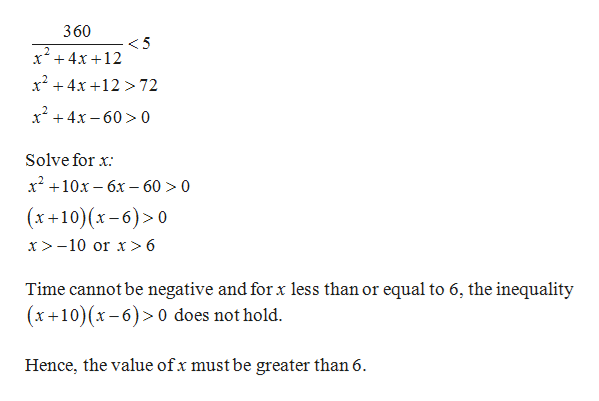help_outlineImage Transcriptionclose360 <5 x4x12 x4x1 72 x+4x-60>0 Solve for x х? +10х — 6х - 60 > 0 (x+10) (x-6)0 x> -10 or x > 6 Time cannot be negative and for x less than or equal to 6, the inequality (x+10) (x-6)> 0 does not hold. Hence, the value ofx must be greater than 6 fullscreen

### Want to see the full answer?

See Solution

#### Want to see this answer and more?

Solutions are written by subject experts who are available 24/7. Questions are typically answered within 1 hour.*

See Solution
*Response times may vary by subject and question.
Tagged in

### Algebra